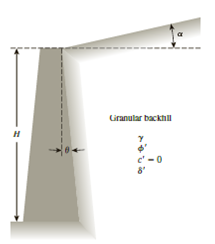Chapter 14, Problem 14.9PPrinciples of Geotechnical Enginee...

9th Edition
Braja M. Das + 1 other
ISBN: 9781305970939

Solutions

Chapter
SectionPrinciples of Geotechnical Enginee...

9th Edition
Braja M. Das + 1 other
ISBN: 9781305970939
Textbook Problem

Redo Problem 14.8 when θ = 10° and α = 0°.14.8 Refer to Figure 14.19. Given: θ = 0, α = 0°, H = 5 m, γ = 15 kN/m3, ϕ ′ = 30 ° , and δ ′ = 15 ° . Estimate the passive force, Pp, per unit length of the wall using Caquot and Kerisel’s solution (Figure 14.6 and Table 14.6).Figure 14.19

To determine

Find the passive force Pp per unit length of the wall using Caquot and Kerisel’s solution.

Explanation

Given information:

The height of the retaining wall is 5.0 m.

The soil friction angle ϕ is 30°.

The angle of wall friction δ is 15°.

The given angle θ value is 10°.

The given angle α value is 0°.

The unit weight γ of the granular backfill is 15kN/m3.

Calculation:

Determine the height H1 using the relation.

H1=Hcosθ

Substitute 5.0 m for H and 10° for θ.

H1=5.0cos10°=5.077m

Calculate the passive force Pp per unit length of the wall using the Caquot and Kerisel’s solution.

Pp=12γH12Kp (1)

Here, Kp is the passive earth pressure coefficient.

Refer Figure 14.6 “Caquot and Kerisel’s solution for Kp” in the text book.

Take the passive earth pressure coefficient Kp for the soil having friction angle of 30° and the angle of 10° as 4

Still sussing out bartleby?

Check out a sample textbook solution.

See a sample solution

The Solution to Your Study Problems

Bartleby provides explanations to thousands of textbook problems written by our experts, many with advanced degrees!

Get Started

What is a relational diagram? Give an example.

Database Systems: Design, Implementation, & Management

The force system shown can be replaced with a single, equivalent couple CR. Determine CR.

International Edition---engineering Mechanics: Statics, 4th Edition

What is interpass temperature?

Welding: Principles and Applications (MindTap Course List)